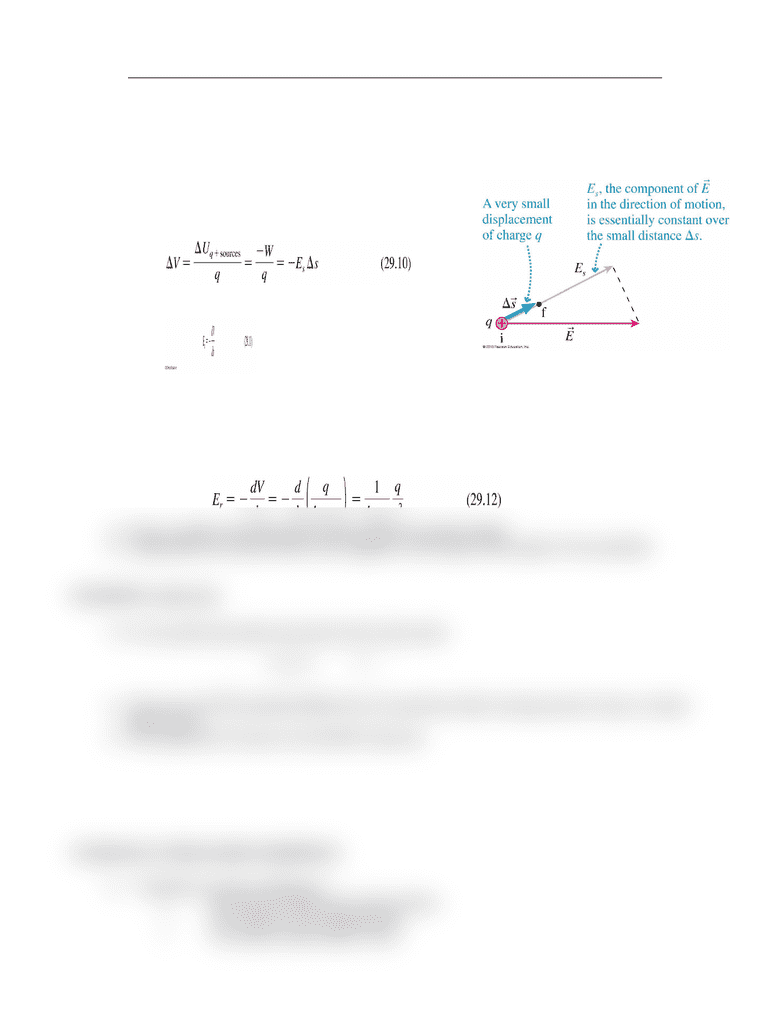# PHYS 142 Lecture Notes - Lecture 10: Equipotential, Electric Field

78 views2 pages
School
Department
CoursePHYS 142: Electromagnetism and Optics - Lecture 10: Potential and Field Part 2
Finding the Electric Field from the Potential:
The figure shows two points i and f separated by
a small distance Δs.
The work done by the electric field as a small
charge q moves from i to f is W = FsΔs = qEsΔs.
The potential difference between the points is:
The electric field in the s-direction is
Es = − ΔVs. In the limit Δs → 0:
Suppose we knew the potential of a point charge to be V = q/4π
0
r but didn’t remember
the electric field.
Symmetry requires that the field point straight outward from the charge, with only a radial
component Er.
If we choose the s-axis to be in the radial direction, parallel to E, we find:
This is, indeed, the well-known electric field of a point charge
Graphically, the electric field is the negative of the slope of the graph of the potential.
Kirchhoff’s Loop Law:
For any path that starts and ends at the same point:
The sum of all the potential differences encountered while moving around a loop or closed
path is zero.
This statement is known as Kirchhoff’s loop law.
Conductors in Electrostatic Equilibrium:
Properties of such conductors:
All excess charge sits on the surface.
The surface is an equipotential.
The electric field inside is zero.
Unlock document

This preview shows half of the first page of the document.
Unlock all 2 pages and 3 million more documents.

## Document Summary

Phys 142: electromagnetism and optics - lecture 10: potential and field part 2. The figure shows two points i and f separated by a small distance s. The work done by the electric field as a small charge q moves from i to f is w = fs s = qes s. The potential difference between the points is: The electric field in the s-direction is. Suppose we knew the potential of a point charge to be v = q/4 0 r but didn"t remember. Symmetry requires that the field point straight outward from the charge, with only a radial the electric field. component er. If we choose the s-axis to be in the radial direction, parallel to e, we find: This is, indeed, the well-known electric field of a point charge. Graphically, the electric field is the negative of the slope of the graph of the potential. For any path that starts and ends at the same point:

# Get access

\$10 USD/m
Billed \$120 USD annually
Homework Help
Class Notes
Textbook Notes